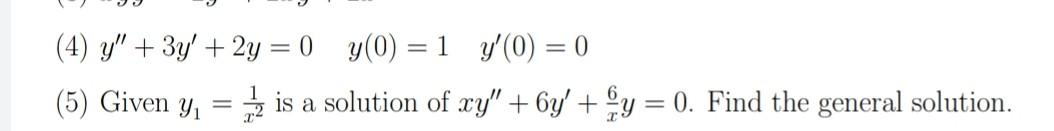### Create an Account

Home / Questions / (4) y" + 3y' + 2y = 0) y(0) =1 y'(0) = 0 (5) Given y, = is a solution of xy" + 6y' + y = 0...

# (4) y" + 3y' + 2y = 0) y(0) =1 y'(0) = 0 (5) Given y, = is a solution of xy" + 6y' + y = 0. Find the general solution.

(4) y" + 3y' + 2y = 0) y(0) =1 y'(0) = 0 (5) Given y, = is a solution of xy" + 6y' + y = 0. Find the general solution.Jun 03 2021 View more View LessSubscribe To Get Solution